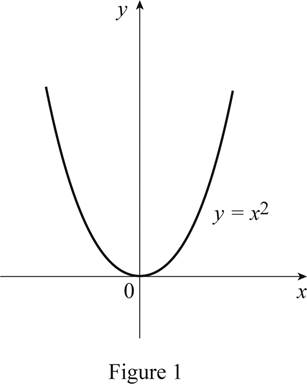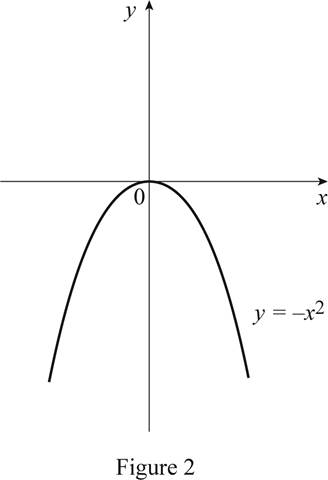# The function y = x 2 by hand without plotting any points and then graph of the function y = − x 2 .### Single Variable Calculus: Concepts...

4th Edition
James Stewart
Publisher: Cengage Learning
ISBN: 9781337687805### Single Variable Calculus: Concepts...

4th Edition
James Stewart
Publisher: Cengage Learning
ISBN: 9781337687805

#### Solutions

Chapter 1.3, Problem 9E
To determine

## To sketch: The function y=x2 by hand without plotting any points and then graph of the function y=−x2 .

Expert Solution

### Explanation of Solution

The standard graph of the function y=x2 is roughly drawn as shown below in Figure 1.Since the function y=x2 is an odd function, reflect the graph y=x2 about the y-axis.

Thus, the graph of y=x2 is shown below in Figure 2.From Figure 2, it is observed that the graph is reflecting the Figure 1 about the y-axis.

### Have a homework question?

Subscribe to bartleby learn! Ask subject matter experts 30 homework questions each month. Plus, you’ll have access to millions of step-by-step textbook answers!